##### Geometry For DummiesMeasuring angles is pretty simple: the size of an angle is based on how wide the angle is open. Here are some points and mental pictures that will help you to understand how angle measurement works.

Degree: The basic unit of measure for angles is the degree.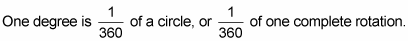A good way to start thinking about the size and degree-measure of angles is by picturing an entire pizza — that’s 360° of pizza. Cut the pizza into 360 slices, and the angle each slice makes is 1°. For other angle measures, see the following list and figure: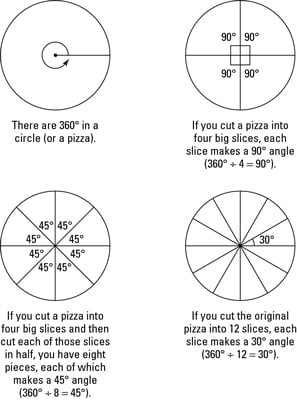• If you cut a pizza into four big slices, each slice makes a 90° angle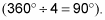• If you cut a pizza into four big slices and then cut each of those slices in half, you get eight pieces, each of which makes a 45° angle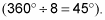• If you cut the original pizza into 12 slices, each slice makes a 30° angle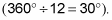So 1/12 of a pizza is 30°, 1/8 is 45°, 1/4 is 90°, and so on.

The bigger the fraction of the pizza, the bigger the angle.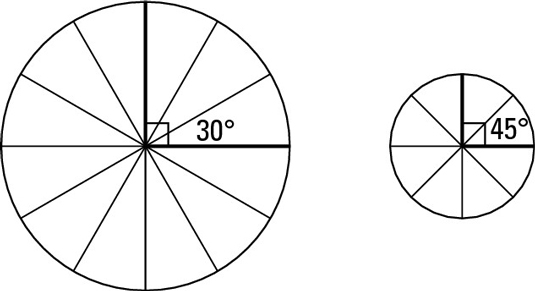The fraction of the pizza or circle is the only thing that matters when it comes to angle size. The length along the crust and the area of the pizza slice tell you nothing about the size of an angle. In other words, 1/6 of a 10-inch pizza represents the same angle as 1/6 of a 16-inch pizza, and 1/8 of a small pizza has a larger angle (45°) than 1/12 of a big pizza (30°) — even if the 30° slice is the one you’d want if you were hungry. You can see this in the above figure.

Another way of looking at angle size is to think about opening a door or a pair of scissors or, say, an alligator’s mouth. The wider the mouth is open, the bigger the angle. As the following figure shows, a baby alligator with its mouth opened wide makes a bigger angle than an adult alligator with its mouth opened less wide, even if there’s a bigger gap at the front of the adult alligator’s mouth.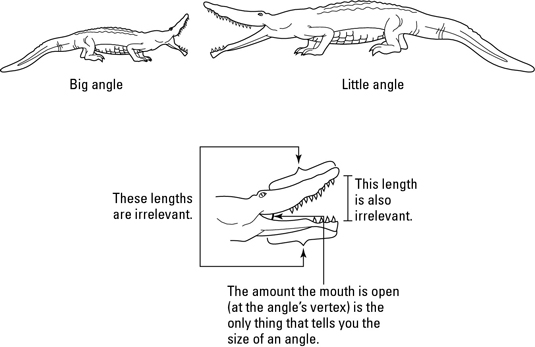An angle’s sides are both rays, and all rays are infinitely long, regardless of how long they look in a figure. The “lengths” of an angle’s sides in a diagram aren’t really lengths at all, and they tell you nothing about the angle’s size. Even when a diagram shows an angle with two segments for sides, the sides are still technically infinitely long rays.

Congruent angles are angles with the same degree measure. In other words, congruent angles have the same amount of opening at their vertices. If you were to stack two congruent angles on top of each other with their vertices together, the two sides of one angle would align perfectly with the two sides of the other angle.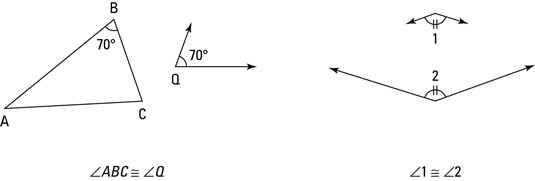You know that two angles are congruent when you know that they both have the same numerical measure (say, they both have a measure of 70°) or when you don’t know their measures but you figure out (or are simply told) that they’re congruent. In figures, angles with the same number of tick marks are congruent to each other, as shown here.